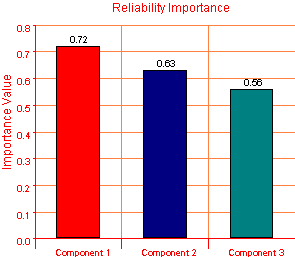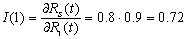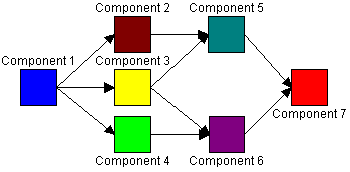# Using Reliability Importance Measures to Guide Component Improvement Efforts

from ReliaSoft
Posted 7/11/2003

The component importance measure is an index of how much or how little an individual component contributes to the overall system reliability. It is useful to obtain the reliability importance value of each component in the system prior to investing resources toward improving specific components. This is done to determine where to focus resources in order to achieve the most benefit from the improvement effort. The reliability importance of a component can be determined based on the failure characteristics of the component and its corresponding position in the system.

Once the reliability of a system has been determined, engineers are often faced with the task of identifying the least reliable components in the system in order to improve the design. For example, in a series system, the least reliable component has the biggest effect on the system reliability. If the reliability of the system needs to be improved, then efforts should first be concentrated on improving the reliability of the component that has the largest effect on reliability. (The cost of improving reliability is not considered in this article. However, this can be done using more complex algorithms available in ReliaSoft’s BlockSim software.) In simple systems such as a series system, it is easy to identify the weak components. However, this becomes more difficult in more complex systems. Therefore, a mathematical approach is needed to provide the means of identifying and quantifying the importance of each component in the system.

Calculating Reliability Importance

The reliability importance, I, of component i in a system of n components is given by:Equation 1

where,

• Rs(t) is the system reliability, and
• Ri(t) is the component reliability.

The value of the reliability importance given by this equation depends both on the reliability of a component and its corresponding position in the system.

Static Reliability Importance

Consider a series system of three components, with reliabilities of 0.7, 0.8, and 0.9 at a given time, t. Using Eqn. (1), the reliability importance in terms of a value for each component can be obtained. The reliability importance values for these components can be calculated using ReliaSoft’s BlockSim. By using the BlockSim plot option and selecting a Static Reliability Importance plot, the graph in Figure 1 can be obtained.Figure 1: Static Reliability Importance Plot

The values shown for each component were obtained using Eqn. (1). The reliability equation for this series system is given by:Taking the partial derivative of Eqn. (2) with respect to R1 yields:Thus the reliability importance of Component 1 is 0.72. The reliability importance values for Components 2 and 3 are obtained in a similar manner.

Time-Dependent Reliability Importance

The reliability importance of a component can be calculated at a specific point in time or over a range of time. In the previous example, time-dependency of the reliability importance was not considered. However, as demonstrated in Eqn. (1), the reliability importance of a component is a function of time. Another way to look at the reliability importance is to generate a plot of Reliability Importance vs. Time. With this plot, the reliability importance of the component as a result of the behavior of its entire failure distribution can be observed rather than the importance relating to just one point on the distribution. For example, Figure 2 illustrates the reliability importance vs. time for a four-component system. In this figure, it can be seen that at 400 hours, Component 4 has a higher reliability importance than Component 1 and at 1200 hours this is reversed. Therefore, the reliability importance measure will vary depending on the time of interest to the analyst.Figure 2: Reliability Importance vs. Time

Application to a Complex System

Consider the system shown in Figure 3. All components have the same reliability of 90% at a given time. The equation for system reliability obtained from BlockSim is given by Eqn. (3).Figure 3: System Reliability Block Diagram and Reliability Importance Plot

Using Eqn. (1), the reliability importance was calculated and the results were plotted in Figure 3. Although the components are identical, their reliability importance is different. This is due to their unique positions within the system. When calculating the reliability importance of a component, its failure properties as well as its system properties are considered.

EXPLORE BY TOPIC